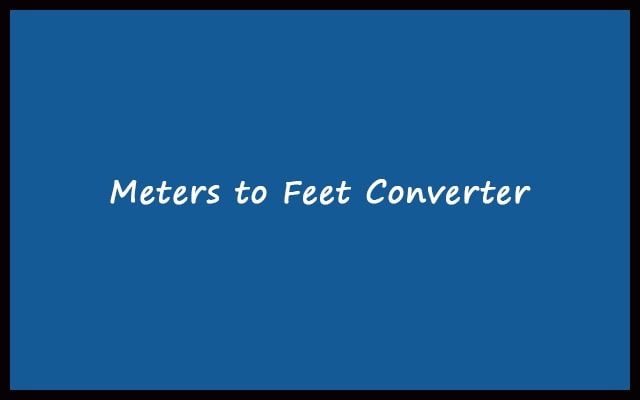# Meters to Feet – m to ft Converter Calculator Online

Meter and Feet are the most commonly heard units of length measurement. Use our meters to feet converter. To change meter values into feet.

Formula: 1 m = 3.28084 feet
 Enter Meters: Feet Output: Feet + Inches Output: All Calculation:
RelatedMeasurement is a very important technique. We find out the length between two points from mathematics classes to daily life. We have measured a lot of things. For measuring it is also necessary to choose a unit. In addition, mensuration units are meters, centimeters, feet, inches, etc. There are some very big units like kilometers and miles. There are some very small units like millimeters and nanometers. Now, it depends on you. Also, on your type of work. If you measured in meters you have to select the measuring unit. Then convert m to ft with our free converter for that. It easily changes meters to feet.

As told earlier, there are different units for measurement. Hence, there are different scales. Scales for recording the measurement. Even more, instruments for measuring are known as scale. You may have used scales for the purpose of mensuration. Ancient people using different techniques to measure. Like stones or crop materials. So, from all these, you get an idea that how important is measurement.

## Why measure m to ft?

There are various reasons for measuring. Some of the daily life need for measuring is as follows:

• For constructing anything. It is important to measure prior to the process. Correct measurements will make a strong structure. Whereas, the structure can collapse if the measurements don’t match with others.
• To know the distance between two places. To know whether the distance is short or long.
• For calculation of area and perimeter we require length and width both. Hence, you need to measure with scale.
• Perfect fitting clothes and shoes are the result of the perfect measurement. A perfect-fitting cloth is very comfortable. Also, enhances look.
• In scientific experiments, different categories of scales are using to measure different kinds of stuff. Microscopic measurements (very small) are also done.

#### Meter Measurement System

Meter is the fundamental and most used unit of length in the metric system. It is also the SI unit of length. 1 meter equals to 100 centimeters. Meter is symbolizing as “m”. Thus we can write 44 meters as 44 m. Both convey the same meaning. The prototype of a meter is actually the distance between two lines on a bar consisting of 90% Platinum and 10% Iridium. This prototype was introduced in the International Bureau of Weights and Measures. There are different other units derived from the meter. For example, centimeter and millimeter for small lengths. Kilometers for larger length and much more.

#### Feet Measurement System

Feet is the plural of the foot. It is the imperial system of measurement. One foot approximately measures the length of a human foot. Nowadays, 1 foot is 30.48 centimeters. Most importantly, Feet or Foot are generally using in English speaking countries. In order to convert your meters to feet, we have created this meters to feet calculator.

## How to convert Meters to Feet?

So, finally, we will learn the mathematics of converting meters into feet. Also, this mathematics is implemented in the converter.

The relationship between meter and feet is:

OR

##### 1 m = (1 / 0.3048) ft = 3.28084 feet

Hence, to find Y feet from Z meter. We will use the equation below:

##### Y = Z / 0.3048

Let’s understand this by seeing some examples.

Example 1: Convert 35 meters to feet

We know that,

Feet = Meter / 0.3048

Therefore,

Feet = 35 / 0.3048 = 114.82939 ft

Hence, 35 meter is equal to 114.82939 ft

Example 2: Convert 0.5892 meters to feet

We know that,
Feet = Meter / 0.3048

So,
Feet = 0.5892 / 0.3048 = 1.9330 ft

As a result, 0.5892 meter is equal to 1.9330 ft

#### Advantages of m to ft converter

1. The calculation for the conversion completes within seconds if you go for the M to Feet converter. Whereas on the other hand, manual calculations will take a lot of time. Hence, save your time and effort. Convert m to ft using the converter.
2. Accuracy of results in the converter is 100%. It is due to the fact that computers never make mistakes like human beings.

#### How to use the Meters to Feet Converter?

1. Firstly, on your device open web browser. Go to the Meters to Feet Converter.
2. Enter your meter value inside the input box.
3. Press the “Convert” button to convert m to ft.
4. As a result, you can find the feet conversion in below box.
5. Lastly, for new conversion just click on the “Reset” button.

#### Meters to feet Conversion Table

Meters (m) Feet (ft)
0.01 m 0.0328 ft
0.1 m 0.3281 ft
1 m 3.2808 ft
2 m 6.5617 ft
3 m 9.8425 ft
4 m 13.1234 ft
5 m 16.4042 ft
6 m 19.6850 ft
7 m 22.9659 ft
8 m 26.2467 ft
9 m 29.5276 ft
10 m 32.8084 ft
20 m 65.6168 ft
30 m 98.4252 ft
40 m 131.2336 ft
50 m 164.0420 ft
60 m 196.8504 ft
70 m 229.6588 ft
80 m 262.4672 ft
90 m 295.2756 ft
100 m 328.0840 ft